## Example Questions

### Example Question #1 : Rational Expressions

Simplify the expression.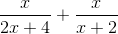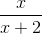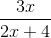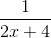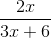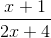Explanation:

To add rational expressions, first find the least common denominator. Because the denominator of the first fraction factors to 2(x+2), it is clear that this is the common denominator. Therefore, multiply the numerator and denominator of the second fraction by 2.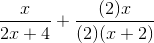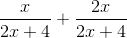This is the most simplified version of the rational expression.

### Example Question #2 : Rational Expressions

Simplify the following: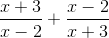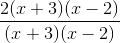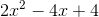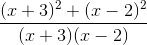Explanation:

To simplify the following, a common denominator must be achieved. In this case, the first term must be multiplied by (x+2) in both the numerator and denominator and likewise with the second term with (x-3).### Example Question #1 : How To Add Rational Expressions With Different Denominators

Choose the answer which best simplifies the following expression: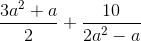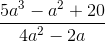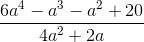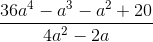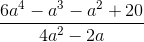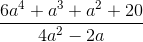Explanation:

To simplify, first multiply both terms by the denominator of the other term over itself: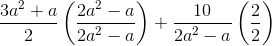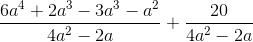Then, you can combine the terms, now that they share a denominator:### Example Question #2 : How To Add Rational Expressions With Different Denominators

Choose the answer which best simplifies the following expression: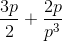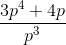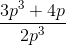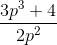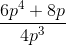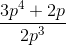Explanation:

To simplify this expression, you have to get both numerators over a common denominator.  The best way to go about doing so is to multiply both expressions by the others denominator over itself: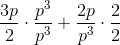Then you are left with: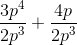Which you can simplify into: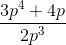From there, you can take out a: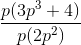### Example Question #3 : How To Add Rational Expressions With Different Denominators

Choose the answer which best simplifies the following expression: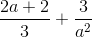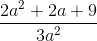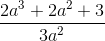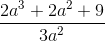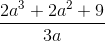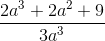Explanation:

To solve this problem, first multiply both terms of the expression by the denominator of the other over itself: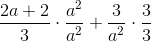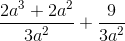Now that both terms have a common denominator, you can add them together:Tired of practice problems?

Try live online GRE prep today.